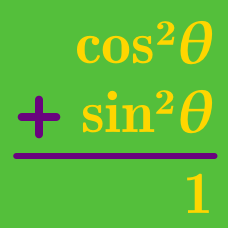Geometry

# Pythagorean Identities: Level 1 Challenges

$\large \dfrac { { \tan }^{ 2 }\theta }{ { \tan }^{ 2 }\theta+ 1 }= \, ?$

Check out the set: 2016 Problems

True or False:

$\large (\cos x - \sin x)(\cos x +\sin x) = \cos^4 x - \sin^4 x$

${\sin}\theta+{\sin}^2\theta= 1$

If the above equation is true, find the value of the expression below

${\cos}^2\theta+{\cos}^4\theta.$

Evaluate \large { \begin{aligned} & \left(12 ^{\sin 24 ^\circ}\right)^{\sin 24 ^\circ} \times \left(12 ^{\sin 66 ^\circ}\right)^{\sin 66 ^\circ} \\ & \times \left(12 ^{\cos 36 ^\circ}\right)^{\cos 36 ^\circ} \times \left(12 ^{\cos 54 ^\circ}\right)^{\cos 54 ^\circ}. \end{aligned}}

Find the value of

$\cos^2 45^{\circ} +\sin^2 45^{\circ}.$

×